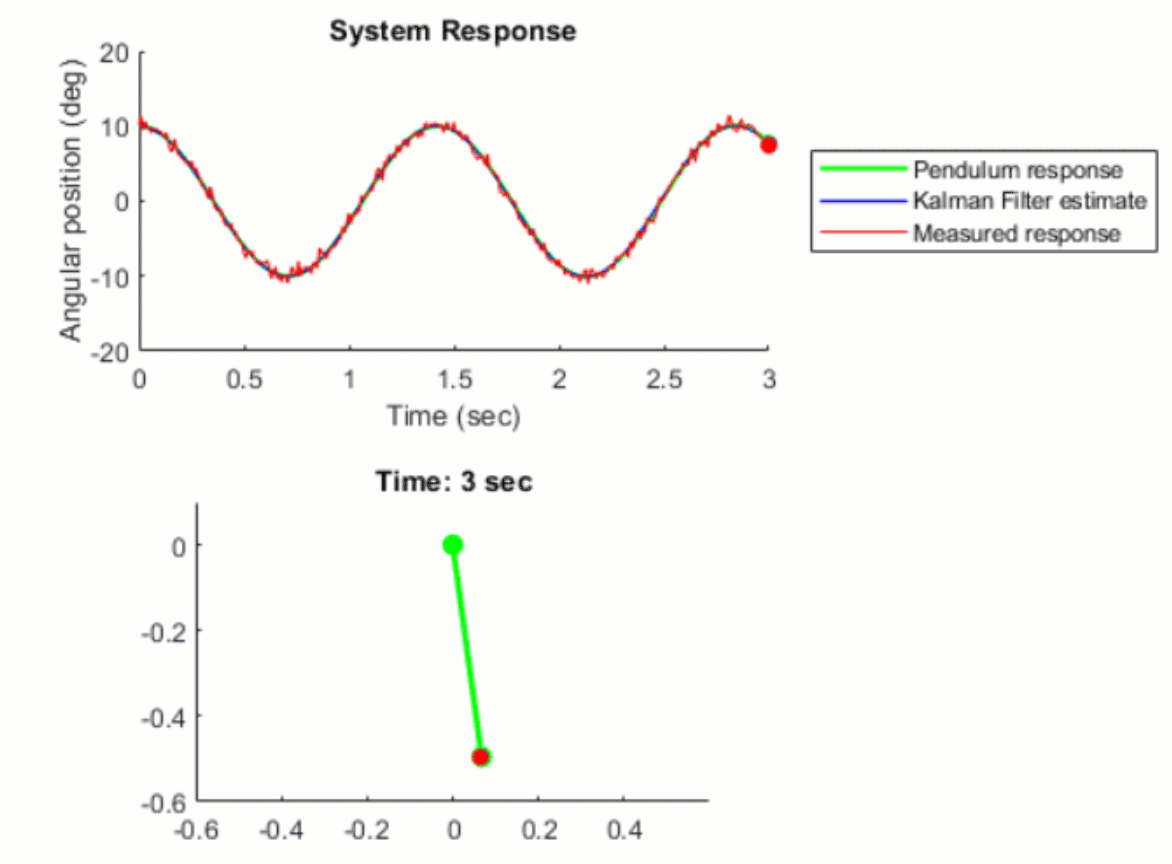## Kalman Filter Virtual Lab

バージョン 1.0.0 (2.7 MB) 作成者:
Kalman Filter virtual laboratory with interactive exercises to study linear and extended Kalman filter design

ダウンロード: 3.3K

GitHub から

# Kalman Filter Virtual LabThe Kalman Filter virtual laboratory contains interactive exercises that let you study linear and extended Kalman filter design for state estimation of a simple pendulum system. The virtual lab lets you visualize pendulum dynamics using 3D simulations and animations (see below).

The lab solutions are available upon instructor request. If you would like to request solutions, find an issue, or have a suggestion, contact us here.## Learning Goals

• Simulate a simple pendulum and visualize its swing motion
• Simulate sensor meaurement and compare measured to actual pendulum response
• Design a Kalman Filter to estimate the angular position of the pendulum
• Design an Extended Kalman Filter to estimate the angular position of the nonlinear pendulum system

## Setup

To get started with the Kalman filter virtual lab, double-click the project.prj file inside MATLAB®. This will set up the parameters required for simulating the pendulum system and open up a live script, where you'll find the instructions for running the interactive exercises to complete the virtual lab.

## Suggested Prework

• Kalman Filter Tech Talks help students gain insights into why engineers use Kalman filters, how Kalman filter state estimation algorithm works and the different types of optimal state estimators such as extended, unscented Kalman filters and particle filters.
• MATLAB Onramp – a free two-hour introductory tutorial that teaches the essentials of MATLAB.
• Simulink Onramp – a free three-hour introductory tutorial that teaches the essentials of Simulink.

## Products

MATLAB, Simulink, Control System Toolbox™, Simscape™, Simscape Multibody™

## Educator Resources

The lab solutions are available upon instructor request. Contact us here if you would like to request solutions or have a question about the lab.

### 引用

Melda Ulusoy (2023). Kalman Filter Virtual Lab (https://github.com/MathWorks-Teaching-Resources/Kalman-filter-virtual-lab/releases/tag/v1.0.0), GitHub. 取得済み .

R2021b と互換性あり
##### プラットフォームの互換性
Windows macOS Linux
##### コミュニティ
Distance Learning コミュニティのその他のファイル

### Community Treasure Hunt

Find the treasures in MATLAB Central and discover how the community can help you!

Start Hunting!

#### models

バージョン 公開済み リリース ノート
1.0.0

この GitHub アドオンでの問題を表示または報告するには、GitHub リポジトリにアクセスしてください。
この GitHub アドオンでの問題を表示または報告するには、GitHub リポジトリにアクセスしてください。